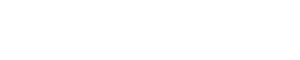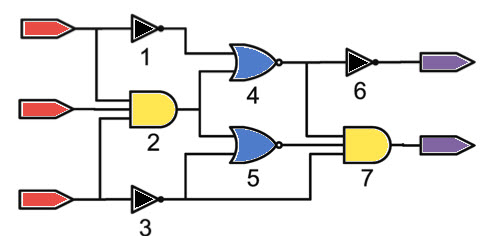Digital Electronics and PLC B.Tech

Course Topics

• Unit 1 - Logic system and Gates
• Logic system and Gates
• Boolean algebra
• Boolean algebra - simplification
• Canonical forms
• Karnaugh Map
• Quine MC cluskey method
• Number systems
• Number system conversions
• Binary codes and BCD codes
• Combinational circuits
• Digital arithmetic circuits
• Multiplexer
• Sequential circuits
• Flip flops
• Flip flop conversions
• Shift registers
• Application of shift registers
• Counters
• Unit 2 -A/D and D/A converter
• Analog to Digital converter
• A/D converter types
• Digital to analog converter
• D/A converter types
• Unit 3 - Digital integrated circuits
• Transistor Transistor Logic
• Cmos Gates
• Semiconductor memories
• Types of memories
• RoM and it’s types
• RAM and it’s types
• Unit 4 -PLC
• PLC block diagram
• PLC block diagram - I/O units
• Working and types of PLC
• PLC module
• USES OF PLC
• BLOCK DIAGRAM OF PLC
• INPUT BLOCK DIAGRAM - PLC
• OUTPUT BLOCK DIAGRAM OF PLC
• CONNECTION OF INPUT TO PLC
• CONNECTION OF OUTPUT TO PLC
• ADVANTAGES AND SIZE OF PLC
• I/O MODULES OF PLC
• I/O CIRCUITS OF PLC
• plc-types of inputs
• PLC-OPERATION
• PLC-PROGRAMMING LANGUAGES
• PLC-TYPES OF PROGRAMMING LANGUAGES
• LADDER LOGIC DIAGRAM FOR LOGIC GATES
• PLC INSTRUCTION
• INPUT,OUTPUT,RELAY,LOGIC INSTRUCTIONS
• PLC-COUNTER INSTRUCTIONS,MATH INSTRUCTIONS
• PLC-DATA COMPARISON & TIMER INSTRUCTIONS
• PLC-JUMP,LABEL AND SEQUENCER INSTRUCTIONS
• PLC-SUBROUTINE INSTRUCTIONS
• PLC-MCR INSTRUCTION
• PLC-COMMUNICATION AND NETWORKS
• PLC-TROUBLESHOOTING
• PLC-SERVICING AND MAINTENANCE
• WORKING PROCEDURE OF SCADA SYSTEMS
• TYPES AND APPLICATION OF SCADA
• MEASURING DEVICES-INTRODUCTION
• Q-METER - DEFINITION , WORKING PRINCIPLE
• Q-METER - CIRCUIT , MEASUREMENTS AND APPLICATION
• LCD-INTRODUCTION,STRUCTURE AND TYPES
• WORKING OF LCD